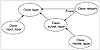# 54.C++ Neural Networks and Fuzzy Logic by Valluru B. Rao M&T Books, IDG Books Worldwide, Inc. ISBN: 1558515526   Pub Date: 06/01/95

#### C++ Classes and Class Hierarchy

So far, you have learned how we address most of the objectives outlined for this program. The only objective left involves the demonstration of some C++ features. In this program we use a class hierarchy with the inheritance feature. Also, we use polymorphism with dynamic binding and function overloading with static binding.

First let us look at the class hierarchy used for this program (see Figure 7.2). An abstract class is a class that is never meant to be instantiated as an object, but serves as a base class from which others can inherit functionality and interface definitions. The layer class is such a class. You will see shortly that one of its functions is set = zero, which indicates that this class is an abstract base class. From the layer class are two branches. One is the input_layer class, and the other is the output_layer class. The middle layer class is very much like the output layer in function and so inherits from the output_layer class.Figure 7.2  Class hierarchy used in the backpropagation simulator.

Function overloading can be seen in the definition of the calc_error() function. It is used in the input_layer with no parameters, while it is used in the output_layer (which the input_layer inherits from) with one parameter. Using the same function name is not a problem, and this is referred to as overloading. Besides function overloading, you may also have operator overloading, which is using an operator that performs some familiar function like + for addition, for another function, say, vector addition.

When you have overloading with the same parameters and the keyword virtual, then you have the potential for dynamic binding, which means that you determine which overloaded function to execute at run time and not at compile time. Compile time binding is referred to as static binding. If you put a bunch of C++ objects in an array of pointers to the base class, and then go through a loop that indexes each pointer and executes an overloaded virtual function that pointer is pointing to, then you will be using dynamic binding. This is exactly the case in the function calc_out(), which is declared with the virtual keyword in the layer base class. Each descendant of layer can provide a version of calc_out(), which differs in functionality from the base class, and the correct function will be selected at run time based on the object’s identity. In this case calc_out(), which is a function to calculate the outputs for each layer, is different for the input layer than for the other two types of layers.

Let’s look at some details in the header file in Listing 7.1:

Listing 7.1 Header file for the backpropagation simulator

` // layer.h            V.Rao, H. Rao // header file for the layer class hierarchy and // the network class #define MAX_LAYERS    5 #define MAX_VECTORS   100 class network; class layer { protected:        int num_inputs;        int num_outputs;        float *outputs;// pointer to array of outputs        float *inputs; // pointer to array of inputs, which                       // are outputs of some other layer        friend network; public:        virtual void calc_out()=0; }; class input_layer: public layer { private: public:        input_layer(int, int);        ~input_layer();        virtual void calc_out(); }; class middle_layer; class output_layer:   public layer { protected:        float * weights;        float * output_errors;    // array of errors at output        float * back_errors;      // array of errors back-propagated        float * expected_values;  // to inputs    friend network; public:        output_layer(int, int);        ~output_layer();        virtual void calc_out();        void calc_error(float &);        void randomize_weights();        void update_weights(const float);        void list_weights();        void write_weights(int, FILE *);        void read_weights(int, FILE *);        void list_errors();        void list_outputs(); }; class middle_layer:   public output_layer { private: public:     middle_layer(int, int);     ~middle_layer();        void calc_error(); }; class network { private:     layer *layer_ptr[MAX_LAYERS];     int number_of_layers;     int layer_size[MAX_LAYERS];     float *buffer;     fpos_t position;     unsigned training; public:     network();     ~network();        void set_training(const unsigned &);        unsigned get_training_value();        void get_layer_info();        void set_up_network();        void randomize_weights();        void update_weights(const float);        void write_weights(FILE *);        void read_weights(FILE *);        void list_weights();        void write_outputs(FILE *);        void list_outputs();        void list_errors();        void forward_prop();        void backward_prop(float &);        int fill_IObuffer(FILE *);        void set_up_pattern(int); }; `C++ Neural Networks and Fuzzy Logic
ISBN: 1558515526
EAN: 2147483647
Year: 1995
Pages: 139

Similar book on Amazon